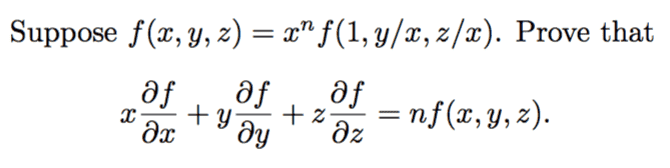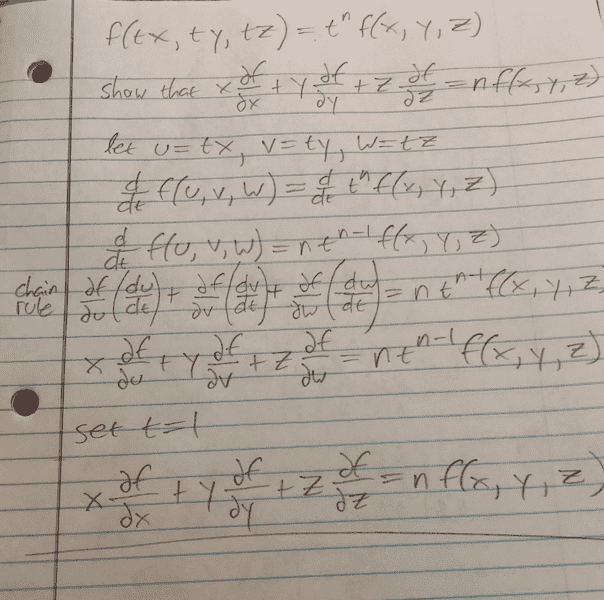# Need help with a calculus III proof

dakota1234
Moved from a technical forum, so homework template missing
I've been working on this one for a little bit, and I know I really just need to use the chain rule to solve it, but I can't seem to figure out how to set it up properly. Probably a dumb question, but I could really use some help on this!#### Attachments

dakota1234
I was able to work out a more generalized version of the proof, but I'm not sure how to apply it#### Attachments

•Delta2
Homework Helper
Gold Member
you are given that ##f(x,y,z)=x^nf(1,y/x,z/x)## (1). If from (1) you can prove that ##f(tx,ty,tz)=t^nf(x,y,z)## (2) then together with the work already done by you , you ll be finished.

Now if you apply (1) for ##x=tx,y=ty,z=tz## what do you get? if you apply it correctly you ll get a result that if you apply (1) again (as it is directly, without substitution) you ll be able to prove (2).

Last edited:
dakota1234
you are given that ##f(x,y,z)=x^nf(1,y/x,z/x)## (1). If from (1) you can prove that ##f(tx,ty,tz)=t^nf(x,y,z)## (2) then together with the work already done by you , you ll be finished.

Now if you apply (1) for ##x=tx,y=ty,z=tz## what do you get? if you apply it correctly you ll get a result that if you apply (1) again (as it is directly, without substitution) you ll be able to prove (2).
I may just be really tired, but can you explain in more detail how "to apply it correctly"? I've tried plugging in the substitutions for x,y,z, since before I even posted on this forum, and I don't understand what I'm doing wrong.

Homework Helper
Gold Member
Ok, let's say that ##f(u,v,w)=u^nf(1,v/u,w/u)## (1)

Applying (1) for ##u=tx,v=ty,w=tz## we get that ##f(tx,ty,tz)=(tx)^nf(1,\frac{ty}{tx},\frac{tz}{tx})=t^nx^nf(1,y/x,z/x)##

Now i have helped too much i think you must be able to see the last step, what is ##x^nf(1,y/x,z/x)## equal to?